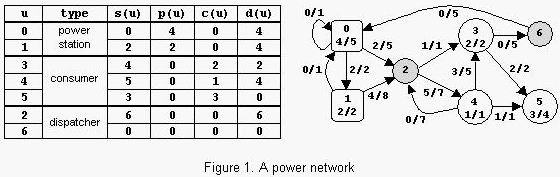# 1977. Power Network

A power network consists of nodes (power stations, consumers and dispatchers) connected by power transport lines. A node $u$ may be supplied with an amount $s(u) \ge 0$ of power, may produce an amount $0 \le p(u) \le pmax(u)$ of power, may consume an amount $0 \le c(u) \le \min {s(u),cmax(u)}$ of power, and may deliver an amount $d(u)=s(u)+p(u)-c(u)$ of power. The following restrictions apply: $c(u)=0$ for any power station, $p(u)=0$ for any consumer, and $p(u)=c(u)=0$ for any dispatcher. There is at most one power transport line $(u,v)$ from a node $u$ to a node $v$ in the net; it transports an amount $0 \leq l(u,v) \leq lmax(u,v)$ of power delivered by $u$ to $v$. Let $Con=\sum_u c(u)$ be the power consumed in the net. The problem is to compute the maximum value of $Con$.An example is in figure 1. The label x/y of power station $u$ shows that $p(u)=x$ and $pmax(u)=y$. The label x/y of consumer $u$ shows that $c(u)=x$ and $cmax(u)=y$. The label x/y of power transport line $(u,v)$ shows that $l(u,v)=x$ and $lmax(u,v)=y$. The power consumed is $Con=6$. Notice that there are other possible states of the network but the value of $Con$ cannot exceed $6$.

### 输入格式

There are several data sets in the input. Each data set encodes a power network. It starts with four integers: $0 \le n \le 100$ (nodes), $0 \le np \le n$ (power stations), $0 \le nc \le n$ (consumers), and $0 \le m \le n^2$ (power transport lines). Follow $m$ data triplets (u,v)z, where $u$ and $v$ are node identifiers (starting from $0$) and $0 \le z \le 1000$ is the value of $lmax(u,v)$. Follow $np$ doublets (u)z, where $u$ is the identifier of a power station and $0 \le z \le 10000$ is the value of $pmax(u)$. The data set ends with $nc$ doublets (u)z, where $u$ is the identifier of a consumer and $0 \le z \le 10000$ is the value of $cmax(u)$. All input numbers are integers. Except the (u,v)z triplets and the (u)z doublets, which do not contain white spaces, white spaces can occur freely in input. Input data terminate with an end of file and are correct.

### 输出格式

For each data set from the input, the program prints on the standard output the maximum amount of power that can be consumed in the corresponding network. Each result has an integer value and is printed from the beginning of a separate line.

### 样例

Input
2 1 1 2 (0,1)20 (1,0)10 (0)15 (1)20
7 2 3 13 (0,0)1 (0,1)2 (0,2)5 (1,0)1 (1,2)8 (2,3)1 (2,4)7
(3,5)2 (3,6)5 (4,2)7 (4,3)5 (4,5)1 (6,0)5
(0)5 (1)2 (3)2 (4)1 (5)4

Output
15
6


### 提示

The sample input contains two data sets. The first data set encodes a network with $2$ nodes, power station $0$ with $pmax(0)=15$ and consumer $1$ with $cmax(1)=20$, and $2$ power transport lines with $lmax(0,1)=20$ and $lmax(1,0)=10$. The maximum value of $Con$ is $15$. The second data set encodes the network from figure 1.

1 人解决，6 人已尝试。

1 份提交通过，共有 6 份提交。

9.9 EMB 奖励。